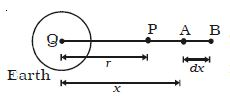# Expression for Gravitational Potential at a Point

Consider a body of mass M at the point C. Let P be a point at a distance r from C. To calculate the gravitational potential at P consider two points A and B. The point A, where the unit mass is placed is at a distance x from C.The gravitational field at A is E = GM/x2

The work done in moving the unit mass from A to B through a small distance dx is du = dv = -E.dx